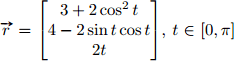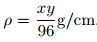# Mass of Ceramic on a Wire

## Homework Statement

A metal wire is given a ceramic coating to protect it against heat. The machine that applies the coating
does not do so very uniformly.

The wire is in the shape of the curveThe density of the ceramic on the wire isUse a line integral to calculate the mass of the ceramic on the wire.

## Homework Equations

∫ f(r,t,ρ) drdtdρ

## The Attempt at a Solution

Here's my 'attempt at a solution':
I tried to make a triple integral, but I don't quite know what to do next.
0π0π0π

## Homework Statement

A metal wire is given a ceramic coating to protect it against heat. The machine that applies the coating
does not do so very uniformly.

The wire is in the shape of the curve
View attachment 103255

The density of the ceramic on the wire is
View attachment 103256

Use a line integral to calculate the mass of the ceramic on the wire.

## Homework Equations

∫ f(r,t,ρ) drdtdρ

## The Attempt at a Solution

Here's my 'attempt at a solution':
I tried to make a triple integral, but I don't quite know what to do next.

First, think about how you would find the length of the metal wire (without the ceramic coating). How would you express that length as a line integral?

http://tutorial.math.lamar.edu/Classes/CalcIII/VectorArcLength.aspx

Once you figure out what a small element of the length of the wire is, then apply the density function of the ceramic coating. How would you calculate the total mass of the ceramic?

... because you are not given a diameter for the wire nor the thickness of the coating, so you have no idea what the volume is.
I would take it that the given density is grams/centimetre, not grams/ cubic metre, and that the curve has units of centimetres.

By the way, you can simplify it by a simple substitution for t.

Ok, so I tried to do it in a CAS. Here is what I got...not sure how right it is though.

x = 3 + 2 (Cos[t]^2)
y = 4 - 2 (Sin[t] Cos[t])
z = 2 t
\[Rho] = (x*y)/96
Dx = D[x, t]
Dy = D[y, t]
Dz = D[z, t]
Integrate[\[Rho]*Sqrt[Dx^2 + Dy^2 + Dz^2], {t, 0, \[Pi]}]

=
(Sqrt \[Pi])/3